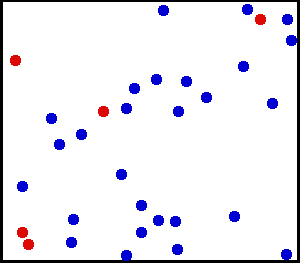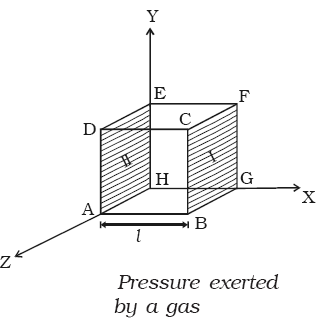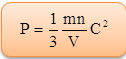×#### Thank you for registering.

One of our academic counsellors will contact you within 1 working day.

Click to Chat

1800-1023-196

+91-120-4616500

CART 0

• 0

MY CART (5)

Use Coupon: CART20 and get 20% off on all online Study Material

ITEM
DETAILS
MRP
DISCOUNT
FINAL PRICE
Total Price: Rs.

There are no items in this cart.
Continue Shopping• Complete Physics Course - Class 11
• OFFERED PRICE: Rs. 2,968
• View Details

```Pressure Exerted by a GasEvery body is made up of molecules. Depending on its nature and temperature, the molecules may possess translatory motion, vibratory motion and rotatory motion about its axis. Each type of motion provides some kinetic energy to the molecules. Heat possessed by a body is the total thermal energy of the body, which is the sum of kinetic energies of all the individual molecules of the body.

Temperature of a body is the degree of hotness or coldness of the body. Heat flows from a body at high temperature to a body at low temperature when they are in contact with each other. Modern concept of temperature follows from zeroth law of thermodynamics.Temperature is the thermal state of the body, that decides the direction of flow of heat.

Temperature is now regarded as one of the fundamental quantities.

Kinetic theory of gases

The founder of modern kinetic theory of heat by common consent is Daniel Bernoulli. But the credit for having established it on a firm mathematical basis is due to Clausius and Maxwell in whose hands it attained the present form.

Postulates of Kinetic theory of gases(a) A gas consists of a very large number of molecules. Each one is a perfectly identical elastic sphere.

(b) The molecules of a gas are in a state of continuous and random motion. They move in all directions with all possible velocities.

(c) The size of each molecule is very small as compared to the distance between them. Hence, the volume occupied by the molecule is negligible in comparison to the volume of the gas.

(d) There is no force of attraction or repulsion between the molecules and the walls of the container.

(e) The collisions of the molecules among themselves and with the walls of the container are perfectly elastic. Therefore, momentum and kinetic energy of the molecules are conserved during collisions.

(f) A molecule moves along a straight line between two successive collisions and the average distance travelled between two successive collisions is called the mean free path of the molecules.

(g) The collisions are almost instantaneous (i.e) the time of collision of two molecules is negligible as compared to the time interval between two successive collisions.

Avogadro number is defined as the number of molecules present in one mole of a substance. It is constant for all the substances. Its value is 6.023 × 1023.

Pressure exerted by a gasThe molecules of a gas are in a state of random motion. They continuously collide against the walls of the container. During each collision, momentum is transfered to the walls of the container. The pressure exerted by the gas is due to the continuous  collision  of  the  molecules against the walls of the container. Due to this  continuous  collision, the  walls experience a continuous force which is equal to the total momentum imparted to the walls per second. The force experienced per unit area of the walls of the container determines the pressure exerted by the gas. Consider a cubic container of side containing n molecules of perfect gas moving with velocities C1, C2, C3  ... Cn as shown in figure. A molecule moving with a velocity C1, will have velocities u1, v1 and w1 as components along the x, y and z axes respectively. Similarly u2, v2 and w2 are the velocity components of the second molecule and so on. Let a molecule P as shown in figure having velocity C  collide against the wall marked I (BCFG) perpendicular to the x-axis. Only the x-component of the velocity of the molecule is relevant for the wall I. Hence momentum of the molecule before collision is mu1 where m is the mass of the molecule. Since the collision is elastic, the molecule will rebound with the velocity u1 in the opposite direction. Hence momentum of the molecule after collision is –mu1.

Change in the momentum of the molecule

= Final momentum - Initial momentum

= –mu1 – mu1 = –2mu1

During each successive collision on face I the molecule must travel a distance 2l from face I to face II and back to face I.

Time taken between two successive collisions is = 2l / u1

∴ Rate of change of momentum = Change in the momentum/Time taken

= – 2mu1/(2l/u1) = -2mu12/2l = – mu12/l

(i.e) Force exerted on the molecule = – mu12/l∴ According to Newton’s third law of motion, the force exerted by the molecule,

= – (– mu12)/l = mu12/l

Force exerted by all the n molecules is

Fx = mu12/l + mu22/l + …...+mun2/l

Pressure exerted by the molecules,

Px = Fx/A

= 1/l2 (mu12/l + mu22/l + …...+mun2/l)

= m/l3 (u12+ u22 + …...+un2)

Similarly, pressure exerted by the molecules along Y and Z axes are,

Py = m/l3 (v12+ v22 + …...+vn2)

Pz = m/l3 (ω12+ ω22 + …...+ωn2)

Since the gas exerts the same pressure on all the walls of the container

Px = Py = Pz = P

P = [Px+Py+Pz]/3

P = [(1/3) (m/l3)] [(u12+ u22 + …...+un2) + (v12+ v22 + …...+vn2) + (ω12+ ω22 + …...+ωn2)]

P = [(1/3) (m/l3)] [(u12 + v12 +ω12) + (u22 + v22 +ω22) +.............+ (un2 + vn2 +ωn2)

P = [(1/3) (m/l3)] [C12+C23+ …...+Cn2]

Here, C12 = (u12 + v12 +ω12)

P = [(1/3) (mn/l3)] [C12+C23+ …...+Cn2/n]

P = (1/3) (mn/V) C2

So,where C is called the root mean square (RMS) velocity, which is defined as the square root of the mean value of the squares of velocities of individual molecules.

That is, C = √[C12+C23+ …...+Cn2/n]

Refer this video to know more about “Pressure Exerted by a Gas”Pressure is associated with collisions of the particles with the container walls.

Gases are made up of molecules: We can treat molecules as point masses that are perfect spheres. Molecules in a gas are very far apart, so that the space between each individual molecule is many orders of magnitude greater than the diameter of the molecule.

Molecules are in constant random motion: There is no general pattern governing either the magnitude or direction of the velocity of the molecules in a gas. At any given time, molecules are moving in many different directions at many different speeds.

The movement of molecules is governed by Newton’s Laws: In accordance with Newton’s First Law, each molecule moves in a straight line at a steady velocity, not interacting with any of the other molecules except in a collision. In a collision, molecules exert equal and opposite forces on one another.

Molecular collisions are perfectly elastic: Molecules do not lose any kinetic energy when they collide with one another.

Gases are made up of particles with no defined volume but with a defined mass. In other words their volume is miniscule compared to the distance between themselves and other molecules.

Gas particles undergo no intermolecular attractions or repulsions. This assumption implies that the particles possess no potential energy and thus their total energy is simply equal to their kinetic energies.

Gas particles are in continuous, random motion.

Collisions between gas particles are completely elastic. In other words, there is no net loss or gain of kinetic energy when particles collide.

The average kinetic energy is the same for all gases at a given temperature, regardless of the identity of the gas. Furthermore, this kinetic energy is proportional to the absolute temperature of the gas.

We can gain a better understanding of pressure (and temperature as well) from the kinetic theory of gases, which assumes that atoms and molecules are in continuous random motion.

Since the assumption is that the particles move in random directions, the average value of velocity squared along each direction must be same.

A gas at pressure P0 is contained in a vessel. If the masses of all the molecules are halved and their speeds doubled, the resulting pressure would be

(a) 4P0                   (b) 2P0

(c) P0                     (d) P0/2

Solution:

We know that, pressure,

P = (1/3) (mn/V) vrms2

That is, P ∝ mvrms2

m is halved and vrms is doubled.

Thus, P will become two times.

Note: In equation (1), m is the mass of one gas molecule and n the total number of gas molecules.

From the above observation we conclude thta, option (b) is correct.Question 1

Which statement is false?

(a) The density of a gas is constant as long as its temperature remains constant.

(b) Gases can be expanded without limit.

(c) Gases diffuse into each other and mix almost immediately when put into the same container.

(d) The molecular weight of a gaseous compound is a non-variable quantity.

(e) Pressure must be exerted on a sample of a gas in order to confine it.

Question 2

Which one of the following statements is not consistent with the kinetic-molecular theory of gases?

(a) Individual gas molecules are relatively far apart.

(b) The actual volume of the gas molecules themselves is very small compared to the volume occupied by the gas at ordinary temperatures and pressures.

(c) The average kinetic energies of different gases are different at the same temperature.

(d) There is no net gain or loss of the total kinetic (translational) energy in collisions between gas molecules.

(e) The theory explains most of the observed behavior of gases at ordinary temperatures and pressures.

Question 3

For a gas, which pair of variables are inversely proportional to each other (if all other conditions remain constant)?

(a) P, T

(b) P, V

(c) V, T

(d) n, V

(e) n, P

Question 4

A real gas most closely approaches the behavior of an ideal gas under conditions of:

(a) high P and low T

(b) low P and high T

(c) low P and T

(d) high P and T

(e) STP

Question 5

A sample of oxygen occupies 47.2 liters under a pressure of 1240 torr at 25oC. What volume would it occupy at 25oC if the pressure were decreased to 730 torr?

(a) 27.8 L

(b) 29.3 L

(c) 32.3 L

(d) 80.2 LQ.1
Q.2
Q.3
Q.4
Q.5

a

c

b

b

d

Related Resources:-

You might like to thermodynamics.

For getting an idea of the type of questions asked, refer the  Previous Year Question Papers.

```### Course Features

• 101 Video Lectures
• Revision Notes
• Previous Year Papers
• Mind Map
• Study Planner
• NCERT Solutions
• Discussion Forum
• Test paper with Video Solution